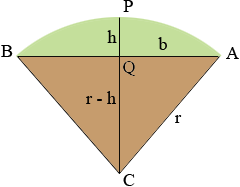SEARCH HOMEMath Central Quandaries & QueriesQuestion from Ivan: What is the formula for figuring out the square footage of arched transom windows & doors?Ivan,

The area of the rectangular part is the width times the height so what remains to bee added is the area of the curved section.I expect the curve is part of a circle so the area can be seen asthe area of the circle sector APBC minus the area of the triangle ABC. In my diagram Q is the midpoint of AB. Let h be the distance from Q to the top of the arch at P and b be the distance from A to Q.

First I need to find the radius of the circle r. Triangle AQC is a right triangle Pythagoras theorem gives us

b2 + (r - h)2 = r2

Simplifying and solving for r gives

r = (b2 + h2)/(2h)                     (*)

Next I need the measure of the angle BCA. The tangent of the angle QCA is b/(r - h) and hence

angle BCA = 2 tan-1[b/(r - h)]

The area of the sector APBC is a fraction of the area of the entire circle (π r2) and the angle BCA is a fraction of 360o. By the symmetry these fractions are the same, that is

area(sector APBC)/ (π r2 ) = (angle BCA)/360o

and hence

area(sector APBC) = 2 π r2 tan-1[b/(r - h)]/360o

The area of the triangle ABC is b(r - h) so finally

area of the transom APB = 2 π r2 tan-1[b/(r - h)]/360o - b(r - h).                     (**)

Thus measure b and h, use expression (*) to find r and then use expression (**) to find the area.

I hope this helps,
HarleyMath Central is supported by the University of Regina and The Pacific Institute for the Mathematical Sciences.